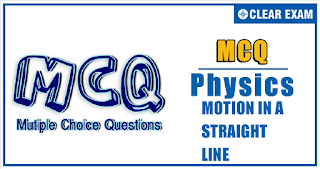## Physics Quiz-3

If an object changes its position with respect to its surroundings with time, then it is called in motion. It is a change in the position of an object over time. Motion in a straight line is nothing but linear motion. As the name suggests, it’s in a particular straight line, thus it can be said that it uses only one dimension.

Q1. A stone thrown upwards with speed u attains maximum height h. Another stone thrown upwards from the same point with speed 2u attains maximum height H. What is the relation between h and H?
•  2h=H
•  3h=H
•  4h=H
•  5h=H
Solution
Maximum height attained ∝ u^2

Q2.A stone is dropped from the top of a tower of height h. After 1 s another stone is dropped from the balcony 20 m below the top. Both reach the bottom simultaneously. What is the value of h? Take g=10 ms^(-2)
•  3125 m
•  312.5 m
•  31.25 m
•  25.31 m
Solution
h=1/2 gt^2 and h-20=1/2 g (t-1)^2
solving them, we get t=2.5 s and h=31.25 m

Q3.  On the displacement-time graph, two straight lines make angle 60° and 30°, with time axis as shown in Fig. The ratio of the velocities represented by them is

•  1:2
•  1:3
•  2:1
•  3:1
Solution
Velocity given by OA=tan⁡〖60°=√3〗  ms^(-1)
Velocity give by AB=-tan⁡〖30°=-1/√3  ms^(-1) 〗
Required ratio =√3/(1/√3)=3:1

Q4. A body starts from rest and travels a distance S with uniform acceleration, then moves uniformly a distance 2S uniformly, and finally comes to rest after moving further 5S under uniform retardation. The ratio of the average velocity to maximum velocity is
• 2/5
•  3/5
•  4/7
•  5/7
Solution
Q5.A body is released from the top of a tower of height H m. After 2 s it is stopped and then instantaneously released. What will be its height after next 2 s?

•  (H-5) m
•  (H-10) m
•  (H-20) m
•  (H-40) m
Solution
Distance covered by the object in first 2 s
h_1=1/2  gt^2=1/2×10×2^2=20 m
Similarly, distance covered by the object in next 2 s will also be 20 m, hence the required height =H-20-20=H-40 m

Q6.
 The distance moved by a freely falling body (starting from rest) during 1st , 2nd, 3rd ,…,n^th second of its motion are proportional to
•  Even numbers
•  Odd numbers
• All integral numbers
•  Squares of integral numbers
Solution
The required ratio is 1 : 3 : 5:… so on

Q7.
A point moves in a straight line so that its displacement x metre at time t second is given by x^2=1+r^2. Its acceleration in ms^(-2) at time t second is
•  1/x^3
•  -(t/x^3)
•  1/x-t^2/x^3
•  1/x-1/x^2
Solution

x^2=1+t^2 or  x=(1+t^2 )^(1/2)
dx/dt=1/2  (1+t^2 )^(-1/2) 2t=t(1+t^2 )^(-1/2)
a=(d^2 x)/(dt^2 )=(1+t^2 )^(-1/2)+t(-1/2) (1+t^2 )^(-3/2) 2t
=1/x-t^2/x^3

Q8. The displacement-time graph of a moving particle with constant acceleration is shown in the           figure. The velocity-time graph is given by

Solution
At t=0 slope of the x-t graph is zero; hence, velocity is zero at t=0. As time increases, slope increases in negative direction; hence, velocity increases, slope increases in negative direction. At point ‘1’ , slope changes suddenly from negative to positive value; hence, velocity changes suddenly from negative to positive and then velocity starts decreasing and becomes zero at ‘2’. Option (a) represents all these clearly

Q9.
 When the speed of a car is u, the minimum distance over which it can be stopped is s. If the speed becomes nu, what will be the minimum distance over which it can be stopped during the same time?•  s/n
•  ns
•  s/n^2
•  n^2s

Solution
v^2-u^2=2as,v=0
s∝u^2
When the initial velocity is made n times, the distance over which it can be stopped becomes n^2 times

Q10. Check up only the correct statement in the following:
•  A body has a constant velocity and still it can have varying speed
•  A body has a constant speed but it can  have varying velocity
•  A body having constant speed cannot have any acceleration
•  None of these
Solution
When a body possesses constant velocity, both its magnitude (i.e., speed) and direction must remain constant. On the other hand, if the speed of a body is constant. For example, in uniform circular motion, though the speed of a body remains constant, velocity changes from point to point due to a change in direction. A body moving with a constant speed along a circular path constantly experiences a centripetal acceleration.#### Written by: AUTHORNAME

AUTHORDESCRIPTION## Want to know more

Please fill in the details below:

Name